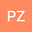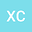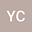The ill-posedness of (non-)periodic travelling wave solution for deformed continuous Heisenberg spin equation
•••• Penghong Zhong,
• Xingfa Chen,
• Ye Chen
Penghong Zhong
Guangdong University of Education

Corresponding Author:zhongpenghong@gdei.edu.cn

Author ProfileXingfa Chen
Guangdong University of Education
Author ProfileYe Chen
Northern Arizona University
Author Profile## Abstract

Based on an equivalent derivative nonlinear Schr\”{o}inger equation, some periodic and non-periodic two-parameter solutions of the deformed continuous Heisenberg spin equation are obtained. These solutions are all proved to be ill-posed by the estimates of Fourier integral in ${H}^{s}_{\mathrm{S}^{2}}$ (periodic solution in ${H}^{s}_{\mathrm{S}^{2}}(\mathbb{T})$ and non-periodic solution in ${H}^{s}_{\mathrm{S}^{2}}(\mathbb{R})$ respectively). If $\alpha \neq 0$, the range of the weak ill-posedness index is \$1